From 09:00 PM Friday, August 7 - 10:30 AM CST Saturday, August 8, ni.com will be undergoing system upgrades that may result in temporary service interruption.

We appreciate your patience as we improve our online experience.

# Frequency Error Measurement

RFmx Bluetooth 19.1 Help

Edition Date: October 2019

Part Number: 377889C-01

»View Product InfoRFmx Bluetooth 19.1 HelpRFmx Bluetooth 20.0 Help

## Frequency Error Measurements

### Basic Rate

Frequency error measurements are performed on Basic Rate packets as specified in section 4.5.8 and 4.5.9 of the Bluetooth Test Specification RF.TS.5.1.0.

1. For each averaging count j (one packet), the following operations are performed.
1. Frequency Error is computed on Preamble. It is recorded as Initial Frequency Error, denoted by ƒ0j2. Frequency error is computed on each 10-bit group in the payload starting from the 2nd bit. These frequency errors are denoted by ƒkj with k = 1, 2,... n is the index of the 10-bit group. The maximum difference between ƒkj and ƒ0j, for all k, is recorded as Peak Frequency Drift.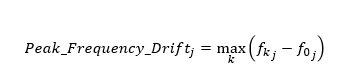3. The maximum difference between ƒk+5j and ƒkj, for all k, is recorded as Peak Frequency Drift Rate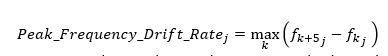2. The value of the Initial Frequency Error that results into the maximum absolute value across all averaging counts is returned as the Initial Frequency Error Maximum.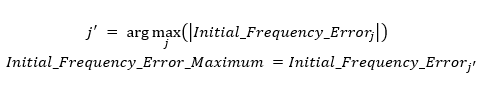3. The value of the Peak Frequency Drift that results into the maximum absolute value across all averaging counts is returned as the Peak Frequency Drift Maximum.4. The value of the Peak Frequency Drift Rate that results into the maximum absolute value across all averaging counts is returned as the Peak Frequency Drift Rate Maximum.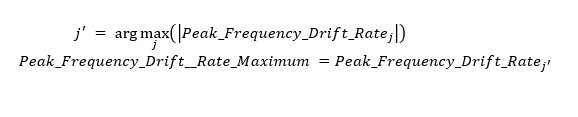### Enhanced Data Rate

Frequency error measurements are performed on Enhanced Data Rate Packets as specified in section 4.5.11 of the Bluetooth Test Specification RF.TS.5.1.0.

1. For each averaging count j (one packet), the following operations are performed.
1. Frequency Error is computed on the packet header. It is recorded as Header Frequency Error and denoted as ωij.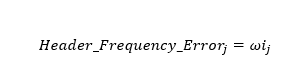2. The Enhanced Data Rate portion of the packet is compensated for ωij. The frequency error is then computed on every 50 Âµs block of EDR portion (include sync, payload Header, EDR payload, trailer and CRC) of the EDR packet. This frequency error is denoted by ω0k,j, where k = 1,2,...n is the index of the 50 Âµs block.
3. The sum of frequency error ωij and ω0k,j, for all k, is recorded as Frequency Error ωij + ω0k,j.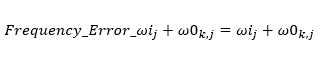4. The maximum value of Frequency ω0k,j, for all k, is recorded as Peak Frequency Error ω0k,j.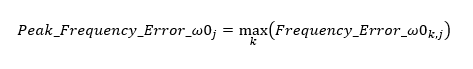5. The maximum value of Frequency Error ωij +ω0k,j, for all k, is recorded as Peak Frequency Error ωij +ω0k,j.2. The value of the Header Frequency Error ωij that results into the maximum absolute value across all averaging counts is returned as the Header Frequency Error ωi Maximum.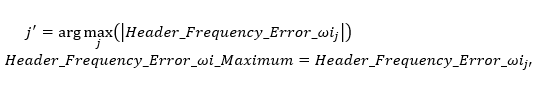3. The value of the Peak Frequency Error ω0j that results into the maximum absolute value across all averaging counts is returned as the Peak Frequency Error ω0 Maximum.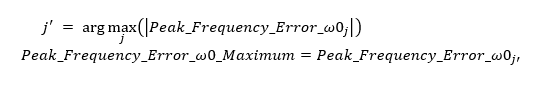4. The value of the Peak Frequency Error ωij + ω0j that results into the maximum absolute value across all averaging counts is returned as the Frequency Error ωi + ω0 Maximum.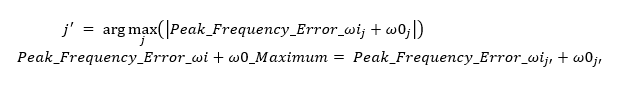### Low Energy (LE)

#### Low Energy Uncoded

##### Low Energy Uncoded without Constant Tone Extension (CTE)

Frequency error measurement are performed on LE packets of symbol rate 1Ms/s and 2Ms/s as specified in section 4.4.4 and 4.4.9 of the Bluetooth Test Specification RF-PHY.TS.5.1.1.

1. For each averaging count j (one packet), the following operations are performed.
1. Frequency error is computed on Preamble. It is recorded as Initial Frequency Error, denoted by ƒ0j.
2. The frequency error is computed starting from the 2nd bit of payload on every 10-bit group of the payload for LE packet with symbol rate 1Ms/s and 20-bit group of the payload for LE packet with symbol rate 2Ms/s. These frequency errors are denoted by ƒnj, where n=1, 2, â€¦, k is the index of the 10-bit or 20-bit payload group of LE packet.
3. The maximum value of ƒnj, for all n, is recorded as Peak Frequency Error.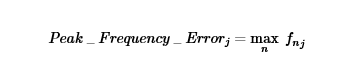4. The difference between ƒ1j and ƒ0j is recorded as Initial Frequency Drift.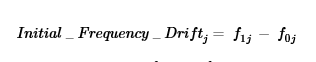2. The maximum difference between ƒnj and ƒ0j, for all n, is recorded as Peak Frequency Drift.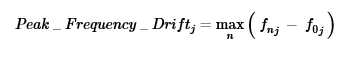3. The maximum difference between ƒnj and ƒn-5j, for n=6, 7,â€¦k, is recorded as Peak Frequency Drift Rate.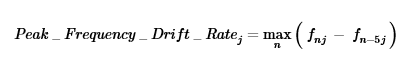• The value of the Peak Frequency Error that results into the maximum absolute value across all averaging counts is returned as the Peak Frequency Error Maximum.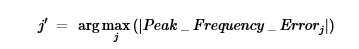• The value of the Initial Frequency Drift that results into the maximum absolute value across all averaging counts is returned as the Initial Frequency Drift Maximum.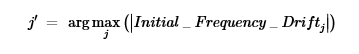• The value of the Peak Frequency Drift that results into the maximum absolute value across all averaging counts is returned as the Peak Frequency Drift Maximum.• The value of the Peak Frequency Drift Rate that results into the maximum absolute value across all averaging count is returned as the Peak Frequency Drift Rate Maximum.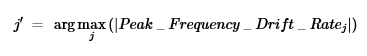##### Low Energy Uncoded With Constant Tone Extension (CTE)

Frequency Error measurement on constant tone extension (CTE) field are performed on LE uncoded packets of symbol rate 1Ms/s and 2Ms/s as specified in section 4.4.13 and 4.4.14 of the Bluetooth Test Specification RF-PHY.TS.5.1.1.

1. For each averaging count j (one packet), the following operations are performed.
1. Frequency error is computed on Preamble. It is recorded as Initial Frequency Error, denoted by ƒ0j.
2. For LE packet of symbol rate 1Ms/s, Frequency error is computed on 16 bits in payload starting from (n+1)th bit of payload where n=((Max_Tx_Length*8)-20). For LE packet of symbol rate 2Ms/s, Frequency Error is computed on 32 bits in payload starting from (n+1)th bit of payload, where n=((Max_Tx_Length*8)-36). First n bits and last 4 bits are discarded. It is recorded as average center frequency, denoted by ƒpj.
3. The average frequency deviation is computed on each 16us unit of CTE, starting from 1st bit of reference period of 16us CTE. The average frequency deviation is denoted by ƒ3maxij, where i=1, 2, â€¦, k is the index of the 16Âµs unit. These frequency errors are denoted by ƒsij.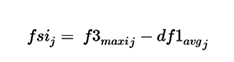For computation of dƒ1avg, refer to the df1 and df2 concept topic.

4. The maximum difference between ƒ3maxij and dƒ1avgj, for all i, is recorded as Peak Frequency Error.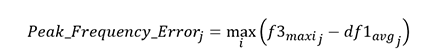5. The difference between ƒs1j and ƒpj is recoded as Initial Frequency Drift.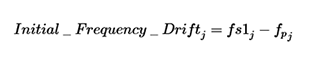6. The maximum difference between ƒs1j and ƒpj for all i, is recorded as Peak Frequency Drift.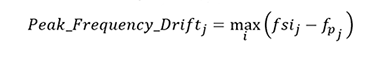7. The maximum difference between ƒs1j and ƒs(i-3)j, for i=4,5,..k, is recorded as Peak Frequency Drift Rate.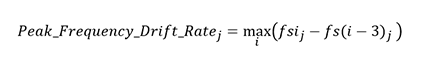2. The value of the Peak Frequency Error that results into the maximum absolute value across all averaging counts is returned as the Peak Frequency Error Maximum.3. The value of the Initial Frequency Drift that results into the maximum absolute value across all averaging counts is returned as the Initial Frequency Drift Maximum.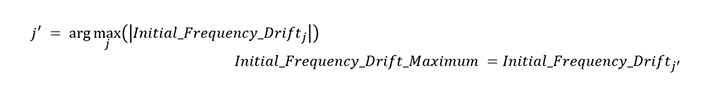4. The value of the Peak Frequency Drift that results into the maximum absolute value across all averaging counts is returned as the Peak Frequency Drift Maximum.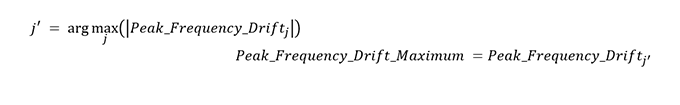5. The value of the Peak Frequency Drift Rate that results into the maximum absolute value across all averaging count is returned as the Peak Frequency Drift Rate Maximum.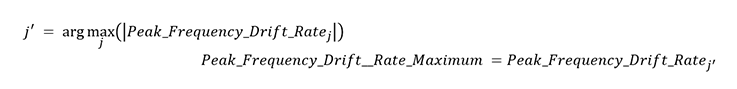#### Low Energy Coded (S=8)

Frequency error measurement are performed on Low Energy Coded (S=8) packets of symbol rate 125Kbps as specified in section 4.4.11 of the Bluetooth Test Specification RF-PHY.TS.5.1.1.

1. For each averaging count j (one packet), the following operations are performed.
1. Frequency error is computed on each group of 16 symbol in preamble field starting at 3rd symbol. The last 14 symbols of preamble are discarded. These frequency errors are recorded as ƒ0j, ƒ1j, ƒ2j, ƒ3j.
2. Frequency error is computed on each 16-symbol group, starting at 27th symbol in the payload. These frequency errors are denoted by ƒnj, where n=4,5,â€¦,k is the index of the 16-symbol group.
3. The maximum value of ƒnj, for all n, is recorded as Peak Frequency Error.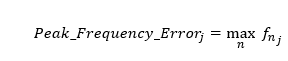4. The maximum difference between ƒnj and ƒ0j, for all n, is recorded as Peak Frequency Drift.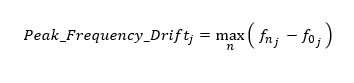5. The maximum difference between ƒnj and ƒn-3j for n=3,4,â€¦,k, is recorded Peak Frequency Drift Rate.2. The value of the Peak Frequency Error that results into the maximum absolute value across all averaging counts is returned as the Peak Frequency Error Maximum.3. The value of the Peak Frequency Drift that results into the maximum absolute value across all averaging counts is returned as the Peak Frequency Drift Maximum.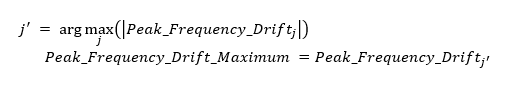4. The value of the Peak Frequency Drift Rate that results into the maximum absolute value across all averaging counts is returned as the Peak Frequency Drift Rate Maximum.